Sunday , May 26 2019
Recent Post
Home / Advance Maths Tricks / Circle & Its Tangent

# Circle & Its TangentNABARD Grade A & B Online Course Get Course Now 150+ RRB NTPC Previous Solved Papers PDF Free Download NowRBI Grade B Complete Online Course Register Today RRB JE Free Study Material PDF Books Free Download Now RRB NTPC Free Study Material PDF Free Download NowAll Exam Free Study Group (Free Videos,Pdf,Mock etc) Join Right Now

# Circle & Its Tangent

Tangent

From Latin: tangere “to touch,”
A line that contacts an arc or circle at only one point.
Try this Drag the orange dot. The blue line will always remain a tangent to the circle.

The blue line in the figure above is called the “tangent to the circle c”.    Another way of saying it is that the blue line is “tangential” to the circle c. (Pronounced “tan-gen-shull”).

The line barely touches the circle at a single point. If the line were closer to the center of the circle, it would cut the circle in two places and would then be called a secant. In fact, you can think of the tangent as the limit case of a secant. As the secant line moves away from the center of the circle, the two points where it cuts the circle eventually merge into one and the line is then the tangent to the circle.

As can be seen in the figure above, the tangent line is always at right angles to the radius at the point of contact.

###Register For NABARD Grade A & B CourseClick Here

## Tangents to two circles

Given two circles, there are lines that are tangents to both of them at the same time.

If the circles are separate (do not intersect), there are four possible common tangents: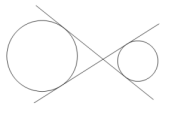Two external tangents Two internal tangents

If the two circles touch at just one point, there are three possible tangent lines that are common to both: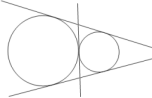If the two circles touch at just one point, with one inside the other, there is just one line that is a tangent to both:If the circles overlap – i.e. intersect at two points, there are two tangents that are common to both:If the circles lie one inside the other, there are no tangents that are common to both. A tangent to the inner circle would be a secant of the outer circle.## Other definitions

• In trigonometry, the tangent of an angle in a right triangle is the ratio of the opposite side to the adjacent side.
• In calculus, a line is a tangent to a curve if, at the single point of contact, it has the same slope as the curve.

## Tangents through an external point

Geometry construction using a compass and straightedge

This page shows how to draw the two possible tangents to a given circle through an external point with compass and straightedge or ruler. This construction assumes you are already familiar with Constructing the Perpendicular Bisector of a Line Segment.

## Proof

This is the same drawing as the last step in the above animation with lines OJ and JM added.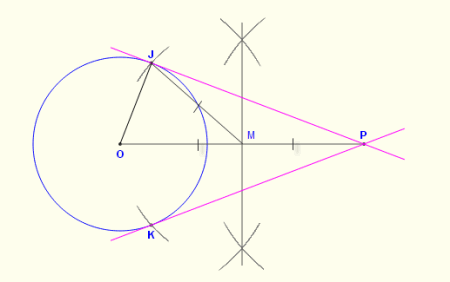Argument Reason
1 OM = MP = JM M was constructed as the midpoint of OP and JM=OM because JM was constructed with compass width set from MO
2 JMO is an isosceles triangle JM=OM from (1)
3 ∠JMO = 180–2(∠OJM) Interior angles of a triangle add to 180°. Base angles of isosceles triangles are equal.
4 JMP is an isosceles triangle JM=MP from (1)
5 ∠JMP = 180–2(∠MJP) Interior angles of a triangle add to 180°. Base angles of isosceles triangles are equal.
6 ∠JMP + ∠JMO = 180 ∠JMP and ∠JMO form a linear pair
7 ∠OJP is a right angle Substituting (3) and (5) into (6):

(180–2∠MJP) + (180–2∠OJM) = 180

Remove parentheses and subtract 360 from both sides:

–2∠MJP –2∠OJM = –180

Divide through by –2::

∠MJP + ∠OJM = 90

8 JP is a tangent to circle O and passes through P JP is a tangent to O because it touches the circle at J and is at right angles to a radius at the contact point.
p KP is a tangent to circle O and passes through P As above but using point K instead of J

Tangent to a circle at a point

Geometry construction using a compass and straightedge

This shows how to construct the tangent to a circle at a given point on the circle with compass and straightedge or ruler. It works by using the fact that a tangent to a circle is perpendicular to the radius at the point of contact. It first creates a radius of the circle, then constructs a perpendicular to the radius at the given point.

## Printable step-by-step instructions

The above animation is available as a printable step-by-step instruction sheet, which can be used for making handouts or when a computer is not available.

## Proof

The image below is the final drawing above.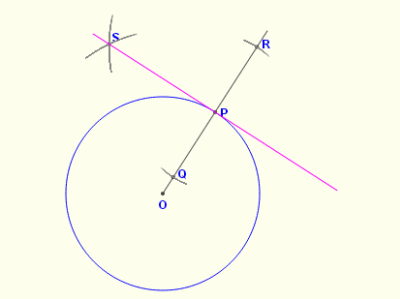Argument Reason
1 Line segment OR is a radius of the circle O. It is a line from the center to the given point P on the circle.
2 SP is perpendicular to OR By construction, SP is the perpendicular to OR at P.
3 SP is the tangent to O at the point P The tangent line is at right angles to the radius at the point of contact.

External tangents to two given circles

Geometry construction using a compass and straightedge

This page shows how to draw one of the two possible external tangents common to two given circles with compass and straightedge or ruler. This construction assumes you are already familiar with Constructing the Perpendicular Bisector of a Line Segment.

## How it works

The figure below is the final construction with the line PJ added.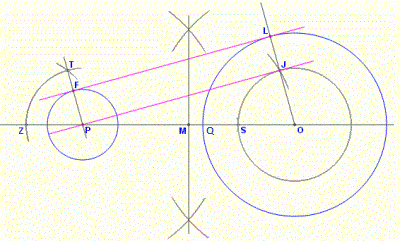The construction has three main steps:

1. The circle OJS is constructed so its radius is the difference between the radii of the two given circles. This means that JL = FP.
2. We construct the tangent PJ from the point P to the circle OJS. This is done using the method described in Tangents through an external point.
3. The desired tangent FL is parallel to PJ and offset from it by JL. Since PJLF is a rectangle, we need the best way to construct this rectangle. The method used here is to construct PF parallel to OL using the “angle copy” method as shown in Constructing a parallel through a point

As shown below, there are two such tangents, the other one is constructed the same way but on the bottom half of the circles.## Proof

This is the same drawing as the last step in the above animation with line PJ added.Argument Reason
1 PJ is a tangent to the inner circle O at J. By construction.
2 FP is parallel to LJ By construction.
3 FP = LJ QS was set from the radius of circle P in construction steps 2 and 3.
4 FPJL is a rectangle
• FP is parallel to and equal to LJ from (2) and (3).
• ∠PJL = ∠PJO = 90° (a tangent is at right angles to radius)
5 ∠FLJ = ∠LFP = 90° Interior angles of rectangles are 90° (4)
6 FL is a tangent to circle O and P Touches the circle at one place (F and L), and is at right angles to the radius at the point of contact

# Tangent (tan) function – Trigonometry

In a right triangle, the tangent of an angle is the length of the opposite side divided by the length of the adjacent side.tan x=O/A
Try this Drag any vertex of the triangle and see how the tangent of A and C are calculated.

The tangent function, along with sine and cosine, is one of the three most common trigonometric functions. In any right triangle, the tangent of an angle is the length of the opposite side (O) divided by the length of the adjacent side (A). In a formula, it is written simply as ‘tan’.

tan x=O/A

As an example, let’s say we want to find the tangent of angle C in the figure above (click ‘reset’ first). From the formula above we know that the tangent of an angle is the opposite side divided by the adjacent side. The opposite side is AB and has a length of 15. The adjacent side is BC with a length of 26. So we can write

tan C=15/26

This division on the calculator comes out to 0.577. So we can say “The tangent of C is 0.5776 ” or

tan C=0.577

## Example – using tangent to find a side length

If we look at the general definition –

tan x=O/A

we see that there are three variables: the measure of the angle x, and the lengths of the two sides (Opposite and Adjacent). So if we have any two of them, we can find the third.

In the figure above, click ‘reset’. Imagine we didn’t know the length of the side BC. We know that the tangent of A (60°) is the opposite side (26) divided by the adjacent side AB – the one we are trying to find.

tan 60°=BC/15

From our calculator we find that tan 60° is 1.733, so we can write

1.733=BC/15

Transposing:

BC=15×1.733

which comes out to 26, which matches the figure above.

## The inverse tangent function – arctan

For every trigonometry function such as tan, there is an inverse function that works in reverse. These inverse functions have the same name but with ‘arc’ in front. So the inverse of tan is arctan etc.

When we see “arctan A”, we interpret it as “the angle whose tangent is A”

 tan 60 = 1.733 Means: The tangent of 60 degrees is 1.733 arctan 1.733 = 60 Means: The angle whose tangent is 1.733 is 60 degrees.
 GovernmentAdda Recommends Ixambee Free Mock TestsNABARD Grade A & B Free All India Mock Test Attempt TodaySBI PO Free All India Mock Test Attempt NowRBI Grade B Free All India Mock Test Attempt Now
 FCI Free Study Material PDF BooksFree Download Now SSC JE Free Study Material PDF BooksFree Download Now Download Quant Power Bank (1000+ Pages)Free Download Now Reasoning & GI Booster -Power Bank Book (1200+ Pages)Free Download Now Free Download English Power Bank Free PDFFree Download Now

### About GovernmentAddaWe provide all Govt Jobs Like Banking, SSC, FCI,UPSC, Railways and all other important government exams Study Materials,Tricks,Quizes,Notifications,Videos etc.

## Quant Booster – A Complete Maths Shortcut Book for Banking SSC and Insurance Exams

NABARD Grade A & B Online Course Get Course Now 150+ RRB NTPC Previous Solved …

error: